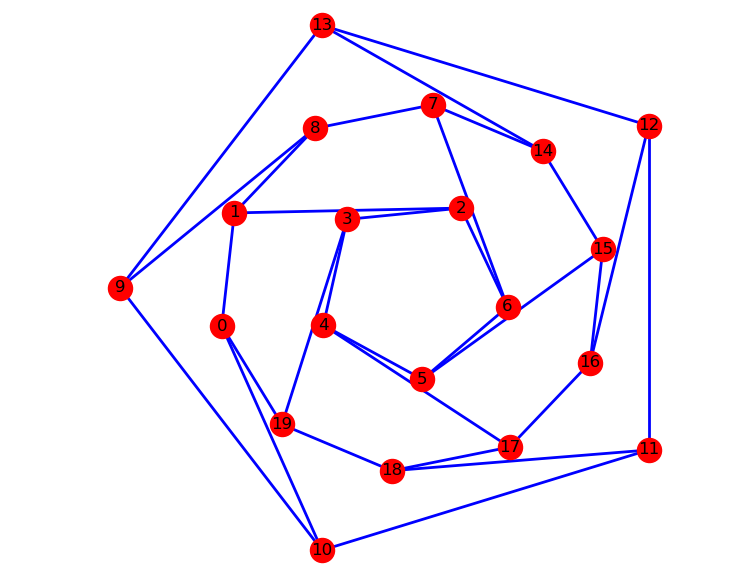# Python小白的数学建模课-15.图论基本概念

• 图论中所说的图，不是图形图像或地图，而是指由顶点和边所构成的图形结构。
• 图论不仅与拓扑学、计算机数据结构和算法密切相关，而且正在成为机器学习的关键技术。
• 本系列结合数学建模的应用需求，来介绍 NetworkX 图论与复杂网络工具包的基本功能和典型算法。
• 『Python小白的数学建模课 @ Youcans』带你从数模小白成为国赛达人。

## 1. 图论

### 1.2 NetworkX 工具包

NetworkX 是基于 Python 语言的图论与复杂网络工具包，用于创建、操作和研究复杂网络的结构、动力学和功能。

NetworkX 可以以标准和非标准的数据格式描述图与网络，生成图与网络，分析网络结构，构建网络模型，设计网络算法，绘制网络图形。

NetworkX 提供了图形的类、对象、图形生成器、网络生成器、绘图工具，内置了常用的图论和网络分析算法，可以进行图和网络的建模、分析和仿真。

NetworkX 的功能非常强大和庞杂，所涉及内容远远、远远地超出了数学建模的范围，甚至于很难进行系统的概括。本系列结合数学建模的应用需求，来介绍 NetworkX 图论与复杂网络工具包的基本功能和典型算法。NetworkX 的官网和文档

## 2、图、顶点和边的创建与基本操作

Networkx 支持创建简单无向图、有向图和多重图；内置许多标准的图论算法，节点可为任意数据；支持任意的边值维度，功能丰富，简单易用。

### 2.1 图的基本概念

• 图（Graph）：图是由若干顶点和连接顶点的边所构成关系结构。
• 顶点（Node）：图中的点称为顶点，也称节点。
• 边（Edge）：顶点之间的连线，称为边。
• 平行边（Parallel edge）：起点相同、终点也相同的两条边称为平行边。
• 循环（Cycle）：起点和终点重合的边称为循环。
• 有向图（Digraph）：图中的每条边都带有方向，称为有向图。
• 无向图（Undirected graph）：图中的每条边都没有方向，称为无向图。
• 赋权图（Weighted graph）：图中的每条边都有一个或多个对应的参数，称为赋权图。该参数称为这条边的权，权可以用来表示两点间的距离、时间、费用。
• 度（Degree）：与顶点相连的边的数量，称为该顶点的度。

### 2.2 图、顶点和边的操作

Networkx很容易创建图、向图中添加顶点和边、从图中删除顶点和边，也可以查看、删除顶点和边的属性。

#### 2.2.1 图的创建

Graph() 类、DiGraph() 类、MultiGraph() 类和 MultiDiGraph() 类分别用来创建：无向图、有向图、多图和有向多图。定义和例程如下：

class Graph(incoming_graph_data=None, **attr)

import networkx as nx  # 导入 NetworkX 工具包

# 创建 图
G1 = nx.Graph()  # 创建：空的 无向图
G2 = nx.DiGraph()  #创建：空的 有向图
G3 = nx.MultiGraph()  #创建：空的 多图
G4 = nx.MultiDiGraph()  #创建：空的 有向多图


#### 2.2.2 顶点的添加、删除和查看

Graph.remove_node(n)
Graph.remove_nodes_from(nodes)

# 顶点(node)的操作
# 向图中添加顶点
G1.add_node(1)  # 向 G1 添加顶点 1
G1.add_node(1, name='n1', weight=1.0)  # 添加顶点 1，定义 name, weight 属性
G1.add_node(2, date='May-16') # 添加顶点 2，定义 time 属性
G1.add_nodes_from([3, 0, 6], dist=1)  # 添加多个顶点，并定义属性
G1.add_nodes_from(range(10, 15))  # 向图 G1 添加顶点 10～14

# 查看顶点和顶点属性
print(G1.nodes())  # 查看顶点列表
# [1, 2, 3, 0, 6, 10, 11, 12, 13, 14]
print(G1._node)  # 查看顶点属性
# {1: {'name': 'n1', 'weight': 1.0}, 2: {'date': 'May-16'}, 3: {'dist': 1}, 0: {'dist': 1}, 6: {'dist': 1}, 10: {}, 11: {}, 12: {}, 13: {}, 14: {}}

# 从图中删除顶点
G1.remove_node(1)  # 删除顶点
G1.remove_nodes_from([1, 11, 13, 14])  # 通过顶点标签的 list 删除多个顶点
print(G1.nodes())  # 查看顶点
# [2, 3, 0, 6, 10, 12]  # 顶点列表


#### 2.2.3 边的添加、删除和查看

# 边(edge)的操作
# 向图中添加边
G1.add_edge(0,10, weight=2.7)  # 向 G1 添加边，并设置边的属性
print(G1.nodes())  # 查看顶点
# [2, 3, 0, 6, 10, 12, 1, 5, 7]  # 自动添加了图中没有的顶点

# 从图中删除边
G1.remove_edge(0,1)  # 从图中删除边 0-1
G1.remove_edges_from([(2,3),(1,5),(6,7)])  # 从图中删除多条边

# 查看 边和边的属性
print(G1.edges)  # 查看所有的边
[(2, 1), (3, 6), (0, 10), (6, 12), (10, 5)]
print(G1.get_edge_data(1,2))  # 查看指定边的属性
# {'weight': 3.6}
print(G1)  # 查看指定边的属性
# {'weight': 3.6}
print(G1.edges(data=True))  # 查看所有边的属性
# [(2, 1, {'weight': 3.6}), (3, 6, {}), (0, 10, {'weight': 2.7}), (6, 12, {'weight': 0.5}), (10, 5, {})]


#### 2.2.4 查看图、顶点和边的信息

# 查看图、顶点和边的信息
print(G1.nodes)  # 返回所有的顶点 [node1,...]
# [2, 3, 0, 6, 10, 12, 1, 5, 7]
print(G1.edges)  # 返回所有的边 [(node1,node2),...]
# [(2, 1), (3, 6), (0, 10), (6, 12), (10, 5)]
print(G1.degree)  # 返回各顶点的度 [(node1,degree1),...]
# [(2, 1), (3, 1), (0, 1), (6, 2), (10, 2), (12, 1), (1, 1), (5, 1), (7, 0)]
print(G1.number_of_nodes())  # 返回顶点的数量
# 9
print(G1.number_of_edges())  # 返回边的数量
# 5
print(G1)  # 返回与指定顶点相邻的所有顶点的属性
# {0: {'weight': 2.7}, 5: {}}
# {0: {'weight': 2.7}, 5: {}}
print(G1)  # 返回指定边的属性
# {'weight': 3.6}
# {'weight': 3.6}
print(G1.degree(10))  # 返回指定顶点的度
# 2

print('nx.info:',nx.info(G1))  # 返回图的基本信息
print('nx.degree:',nx.degree(G1))  # 返回图中各顶点的度
print('nx.density:',nx.degree_histogram(G1))  # 返回图中度的分布
print('nx.pagerank:',nx.pagerank(G1))  # 返回图中各顶点的频率分布


### 2.3 图的属性和方法

G.has_node(n) 当图 G 中包括顶点 n 时返回 True
G.has_edge(u, v) 当图 G 中包括边 (u,v) 时返回 True
G.number_of_nodes() 返回 图 G 中的顶点的数量
G.number_of_edges() 返回 图 G 中的边的数量
G.number_of_selfloops() 返回 图 G 中的自循环边的数量
G.degree([nbunch, weight]) 返回 图 G 中的全部顶点或指定顶点的度
G.selfloop_edges([data, default]) 返回 图 G 中的全部的自循环边
G.subgraph([nodes]) 从图 G1中抽取顶点[nodes]及对应边构成的子图
union(G1,G2) 合并图 G1、G2
nx.info(G) 返回图的基本信息
nx.degree(G) 返回图中各顶点的度
nx.degree_histogram(G) 返回图中度的分布
nx.pagerank(G) 返回图中各顶点的频率分布

G1.clear() # 清空图G1
nx.add_star(G1, [1, 2, 3, 4, 5], weight=1)  # 添加星形网络：以第一个顶点为中心
# [(1, 2), (1, 3), (1, 4), (1, 5)]
nx.add_path(G1, [5, 6, 8, 9, 10], weight=2)  # 添加路径：顺序连接 n个节点的 n-1条边
# [(5, 6), (6, 8), (8, 9), (9, 10)]
nx.add_cycle(G1, [7, 8, 9, 10, 12], weight=3)  # 添加闭合回路：循环连接 n个节点的 n 条边
# [(7, 8), (7, 12), (8, 9), (9, 10), (10, 12)]
print(G1.nodes)  # 返回所有的顶点 [node1,...]
nx.draw_networkx(G1)
plt.show()

G2 = G1.subgraph([1, 2, 3, 8, 9, 10])
G3 = G1.subgraph([4, 5, 6, 7])
G = nx.union(G2, G3)
print(G.nodes)  # 返回所有的顶点 [node1,...]
# [1, 2, 3, 8, 9, 10, 4, 5, 6, 7]


## 3、图的绘制与分析

### 3.1 图的绘制

draw(G[,pos,ax]) 基于 Matplotlib 绘制 图 G
draw_networkx(G[, pos, arrows, with_labels]) 基于 Matplotlib 绘制 图 G
draw_networkx_nodes(G, pos[, nodelist, . . . ]) 绘制图 G 的顶点
draw_networkx_edges(G, pos[, edgelist, . . . ]) 绘制图 G 的边
draw_networkx_labels(G, pos[, labels, . . . ]) 绘制顶点的标签
draw_networkx_edge_labels(G, pos[, . . . ]) 绘制边的标签

draw(G, pos=None, ax=None, **kwds)

draw_networkx(G, pos=None, arrows=True, with_labels=True, **kwds)

• 'node_size'：指定节点的尺寸大小，默认300
• 'node_color'：指定节点的颜色，默认红色
• 'node_shape'：节点的形状，默认圆形
• ''alpha'：透明度，默认1.0，不透明
• 'width'：边的宽度，默认1.0
• 'edge_color'：边的颜色，默认黑色
• 'style'：边的样式，可选 'solid'、'dashed'、'dotted'、'dashdot'
• 'with_labels'：节点是否带标签，默认True
• 'font_size'：节点标签字体大小，默认12
• 'font_color'：节点标签字体颜色，默认黑色### 3.2 图的分析

NetwotkX 提供了图论函数对图的结构进行分析：

• 子图是指顶点和边都分别是图 G 的顶点的子集和边的子集的图。
• subgraph()方法，按顶点从图 G 中抽出子图。例程如前。

• 如果图 G 中的任意两点间相互连通，则 G 是连通图。
• connected_components()方法，返回连通子图的集合。
G = nx.path_graph(4)
# 连通子图
listCC = [len(c) for c in sorted(nx.connected_components(G), key=len, reverse=True)]
maxCC = max(nx.connected_components(G), key=len)
print('Connected components:{}'.format(listCC))  # 所有连通子图
# Connected components:[4, 3]
print('Largest connected components:{}'.format(maxCC))  # 最大连通子图
# Largest connected components:{0, 1, 2, 3}


• 如果有向图 G 中的任意两点间相互连通，则称 G 是强连通图。
• strongly_connected_components()方法，返回所有强连通子图的列表。
# 强连通
G = nx.path_graph(4, create_using=nx.DiGraph())
# 找出所有的强连通子图
con = nx.strongly_connected_components(G)
print(type(con),list(con))
# <class 'generator'> [{8, 1, 2, 3}, {0}]


• 如果一个有向图 G 的基图是连通图，则有向图 G 是弱连通图。
• weakly_connected_components()方法，返回所有弱连通子图的列表。
# 弱连通
G = nx.path_graph(4, create_using=nx.DiGraph())  #默认生成节点 0,1,2,3 和有向边 0->1,1->2,2->3
con = nx.weakly_connected_components(G)
print(type(con),list(con))
# <class 'generator'> [{0, 1, 2, 3, 7, 8}]


【本节完】